# Adams–Hilton model

Jump to: navigation, search

The Pontryagin algebra(cf. also Pontryagin invariant; Pontryagin class) of a topological spaceis an important homotopy invariant (cf. also Homotopy type). It is, in general, quite difficult to calculate the homologydirectly from the chain complex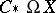. An algorithm that associates to a spacea differential graded algebra whose homology is relatively easy to calculate and isomorphic as an algebra tois therefore of great value.

In 1955, J.F. Adams and P.J. Hilton invented such an algorithm for the class of simply-connected CW-complexes [a1]. Presented here in a somewhat more modern incarnation, due to S. Halperin, Y. Félix and J.-C. Thomas [a5], the work of Adams and Hilton can be summarized as follows.

Letbe a CW-complex such thathas exactly one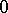-cell and no-cells and such that every attaching mapping is based with respect to the unique-cell of. There exists a morphism of differential graded algebras inducing an isomorphism on homology (a quasi-isomorphism)such thatrestricts to quasi-isomorphisms, where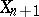denotes the-skeleton of,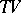denotes the free (tensor) algebra on a free graded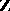-module, and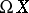is the space of Moore loops on. The morphism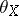is called an Adams–Hilton model ofand satisfies the following properties.is unique up to isomorphism;

if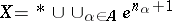, thenhas a degree-homogeneous basis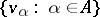such that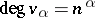;

ifis the attaching mapping of the cell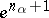, then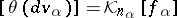. Here,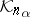is defined so that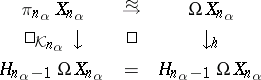commutes, where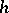denotes the Hurewicz homomorphism (cf. Homotopy group).

The Adams–Hilton model has proved to be a powerful tool for calculating the Pontryagin algebra of CW-complexes. Many common spaces have Adams–Hilton models that are relatively simple and thus well-adapted to computations. For example, with respect to its usual CW-decomposition, the Adams–Hilton model of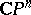is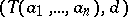, where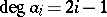and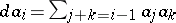.

Given a cellular mappingbetween CW-complexes, it is possible to use the Adams–Hilton model to compute the induced homomorphism of Pontryagin algebras. If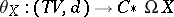and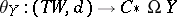are Adams–Hilton models, then there exists a unique homotopy class of morphisms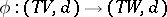such that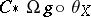is homotopic to. Any representativeof this homotopy class can be said to be an Adams–Hilton model of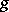. In this context, "homotopy" means homotopy in the category of differential graded algebras (see [a2] or [a5] for more details).

One can say, for example, that an Adams–Hilton model of a cellular co-fibration of CW-complexes, i.e., an inclusion of CW-complexes, is a free extension of differential graded algebras. Furthermore, the Adams–Hilton model of the amalgamated sum of an inclusion of CW-complexes,, and any other cellular mappingis given by the amalgamated sum of the free extension modellingand an Adams–Hilton model of.

Examples of problems to which Adams–Hilton models have been applied to great advantage include the study of the holonomy action in fibrations [a4] and the study of the effect on the Pontryagin algebra of the attachment of a cell to a CW-complex [a3], [a6].